# Excel Formula Evaluator

Excel
If you would like to understand better how some of these complex array formulas work, consider using a handy tool - Formula Evaluator: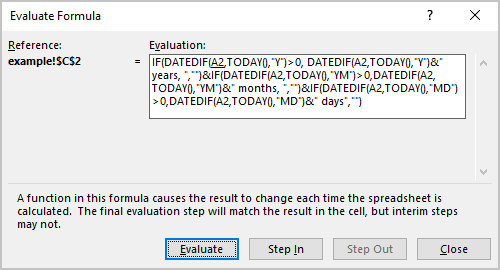Select the cell that contains the formula, and on the Formulas tab, in the Formula Auditing group, click Evaluate Formula: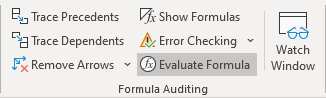In the Evaluate Formula dialog box:

• Click the Evaluate button repeatedly to see the intermediate results as the formula is being calculated. The result of the evaluation is shown in italics: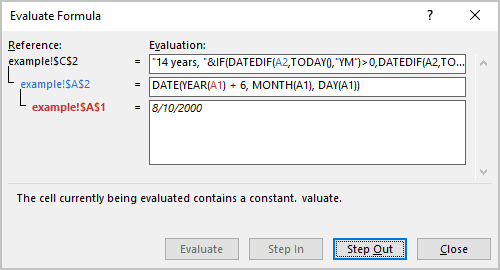• If the underlined part of the formula is a reference to another formula, click the Step In button to display the other formula in the Evaluation box: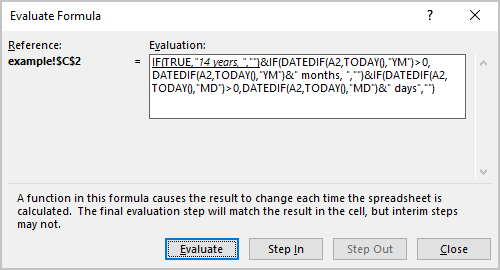• Click the Step Out button to go back to the previous cell and formula: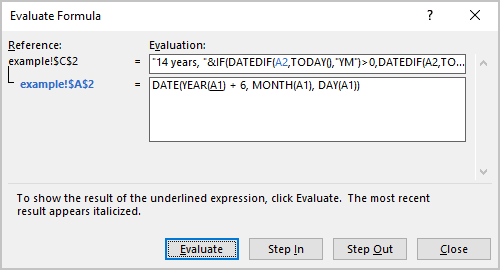• Click the Restart button to see the evaluation again.